# Chapter 4. Naive Bayesian Classification

Remember email several years ago? You probably recall your inbox being full of spam messages ranging from Nigerian princes wanting to pawn off money to pharmaceutical advertisements. It became such a major issue that we spent most of our time filtering spam.

Nowadays, we spend a lot less time filtering spam than we used to, thanks to Gmail and tools like SpamAssassin. Using a method called a Naive Bayesian Classifier, such tools have been able to mitigate the influx of spam to our inboxes. This chapter will explore that topic as well as:

• Bayes’ theorem

• What a Naive Bayesian Classifier is and why it’s called “naive”

• How to build a spam filter using a Naive Bayesian Classifier

###### Note

As noted in Chapter 2, a Naive Bayes Classifier is a supervised and probabalistic learning method. It does well with data in which the inputs are independent from one another. It also prefers problems where the probability of any attribute is greater than zero.

# Using Bayes’ Theorem to Find Fraudulent Orders

Imagine you’re running an online store and lately you’ve been overrun with fraudulent orders. You estimate that about 10% of all orders coming in are fraudulent. In other words, in 10% of orders, people are stealing from you. Now of course you want to mitigate this by reducing the fraudulent orders, but you are facing a conundrum.

Every month you receive at least 1,000 orders, and if you were to check every single one, you’d spend more money fighting fraud than the fraud was costing you in the first place. Assuming that it takes up to 60 seconds per order to determine whether it’s fraudulent or not, and a customer service representative costs around \$15 per hour to hire, that totals 200 hours and \$3,000 per year.

Another way of approaching this problem would be to construct a probability that an order is over 50% fraudulent. In this case, we’d expect the number of orders we’d have to look at to be much lower. But this is where things become difficult, because the only thing we can determine is the probability that it’s fraudulent, which is 10%. Given that piece of information, we’d be back at square one looking at all orders because it’s more probable that an order is not fraudulent!

Let’s say that we notice that fraudulent orders often use gift cards and multiple promotional codes. Using this knowledge, how would we determine what is fraudulent or not—namely, how would we calculate the probability of fraud given that the purchaser used a gift card?

To answer for that, we first have to talk about conditional probabilities.

## Conditional Probabilities

Most people understand what we mean by the probability of something happening. For instance, the probability of an order being fraudulent is 10%. That’s pretty straightforward. But what about the probability of an order being fraudulent given that it used a gift card? To handle that more complicated case, we need something called a conditional probability, which is defined as follows:This definition basically says that the probability of A happening given that B happened is the probability of A and B happening divided by the probability of B. Graphically, it looks something like Figure 4-1.

In our fraud example, let’s say we want to measure the probability of fraud given that an order used a gift card. This would be. Now this works if you know the actual probability of Fraud and Giftcard happening.

At this point, we are up against the problem that we cannot calculate P(Fraud|Giftcard) because that is hard to separate out. To solve this problem, we need to use a trick introduced by Bayes.

## Inverse Conditional Probability (aka Bayes’ Theorem)

In the 1700s, Reverend Thomas Bayes came up with the original research that would become Bayes’ theorem. Pierre-Simon Laplace extended Bayes’ research to produce the beautiful result we know today. Bayes’ theorem is as follows:This is because of the following:This is useful in our fraud example because we can effectively back out our result using other information. Using Bayes’ theorem, we would now calculate:Remember that the probability of fraud was 10%. Let’s say that the probability of gift card use is 10%, and based on our research the probability of gift card use in a fraudulent order is 60%. So what is the probability that an order is fraudulent given that it uses a gift card?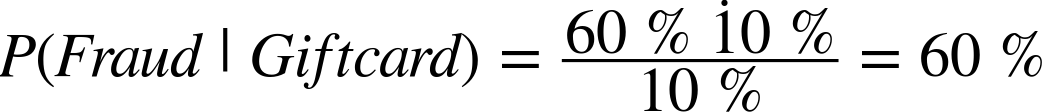The beauty of this is that your work on measuring fraudulent orders is drastically reduced because all you have to look for is the orders with gift cards. Because the total number of orders is 1,000, and 100 of those are fraudulent, we will look at 60 of those fraudulent orders. Out of the remaining 900, 90 used gift cards, which brings the total we need to look at to 150!

At this point, you’ll notice we reduced the orders needing fraud review from 1,000 to 40 (i.e., 4% of the total). But can we do better? What about introducing something like people using multiple promo codes or other information?

# Naive Bayesian Classifier

We’ve already solved the problem of finding fraudulent orders given that a gift card was used, but what about the problem of fraudulent orders given the fact that they have gift cards, or multiple promo codes, or other features? How would we go about that?

Namely, we want to solve the problem of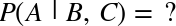. For this, we need a bit more information and something called the chain rule.

## The Chain Rule

If you think back to probability class, you might recall that the probability of A and B happening is the probability of B given A times the probability of A. Mathematically, this looks like P(A ∩ B) = P(B | A)P(A). This is assuming these events are not mutually exclusive. Using something called a joint probability, this smaller result transforms into the chain rule.

Joint probabilities are the probability that all the events will happen. We denote this by using ∩. The generic case of the chain rule is:

P(A1, A2, …, An) = P(A1)P(A2 | A1)P(A3 | A1, A2) … P(An | A1, A2, …, An–1)

This expanded version is useful in trying to solve our problem by feeding lots of information into our Bayesian probability estimates. But there is one problem: this can quickly evolve into a complex calculation using information we don’t have, so we make one big assumption and act naive.

## Naivety in Bayesian Reasoning

The chain rule is useful for solving potentially inclusive problems, but we don’t have the ability to calculate all of those probabilities. For instance, if we were to introduce multiple promos into our fraud example then we’d have the following to calculate: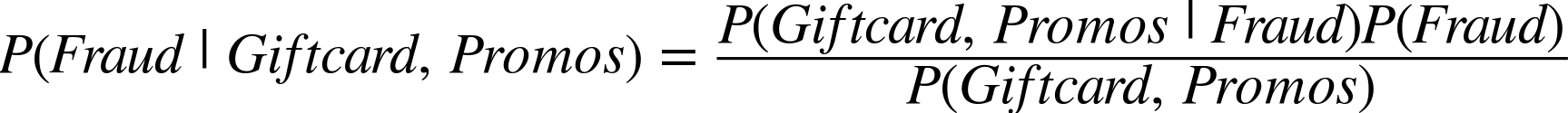Let’s ignore the denominator for now, as it doesn’t depend on whether the order is fraudulent or not. At this point, we need to focus on finding the calculation for P(Giftcard, Promos|Fraud)P(Fraud). If we apply the chain rule, this is equivalent to P(Fraud, Giftcard, Promos).

You can see this by the following:

Now at this point we have a conundrum: how do you measure the probability of a promo code given fraud and gift cards? While this is the correct probability, it really can be difficult to measure—especially with more features coming in. What if we were to be a tad naive and assume that we can get away with independence and just say that we don’t care about the interaction between promo codes and gift cards, just the interaction of each independently with fraud?

In that case, our math would be much simpler:

This would be proportional to our numerator. And, to simplify things even more, we can assert that we’ll normalize later with some magical Z, which is the sum of all the probabilities of classes. So now our model becomes: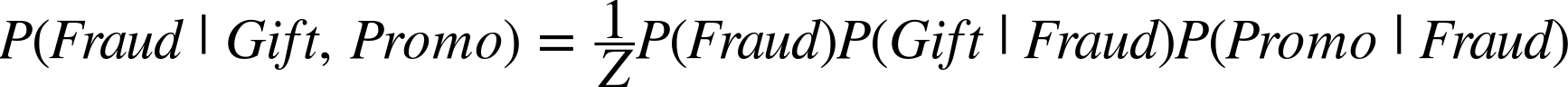To turn this into a classification problem, we simply determine which input—fraud or not fraud—yields the highest probability. See Table 4-1.

Table 4-1. Probability of gift cards versus promos
Fraud Not fraud

60%

10%

Multiple promos used

50%

30%

Probability of class

10%

90%

At this point, you can use this information to determine whether an order is fraudulent based purely on whether it has a gift card present and whether it used multiple promos. The probability that an order is fraudulent given the use of gift cards and multiple promos is 62.5%. While we can’t exactly figure out how much savings this gives you in terms of the number of orders you must review, we know that we’re using better information and making a better judgment.

There is one problem, though: what happens when the probability of using multiple promos given a fraudulent order is zero? A zero result can happen for several reasons, including that there just isn’t enough of a sample size. The way we solve this is by using something called a pseudocount.

## Pseudocount

There is one big challenge with a Naive Bayesian Classifier, and that is the introduction of new information. For instance, let’s say we have a bunch of emails that are classified as spam or ham. We build our probabilities using all of this data, but then something bad happens: a new spammy word, fuzzbolt. Nowhere in our data did we see the word fuzzbolt, and so when we calculate the probability of spam given the word fuzzbolt, we get a probability of zero. This can have a zeroing-out effect that will greatly skew results toward the data we have.

Because a Naive Bayesian Classifier relies on multiplying all of the independent probabilities together to come up with a classification, if any of those probabilities are zero then our probability will be zero.

Take, for instance, the email subject “Fuzzbolt: Prince of Nigeria.” Assuming we strip off of, we have the data shown in Table 4-2.

Table 4-2. Probability of word given spam or ham

Word

Spam

Ham

Fuzzbolt

0

0

Prince

75%

15%

Nigeria

85%

10%

Now let’s assume we want to calculate a score for ham or spam. In both cases, the score would end up being zero because fuzzbolt isn’t present. At that point, because we have a tie, we’d just go with the more common situation, which is ham. This means that we have failed and classified something incorrectly due to one word not being recognized.

There is an easy fix for that: pseudocount. When we go about calculating the probability, we add one to the count of the word. So, in other words, everything will end up being `word_count` + 1. This helps mitigate the zeroing-out effect for now. In the case of our fraud detector, we would add one to each count to ensure that it is never zero.

So in our preceding example, let’s say we have 3,000 words. We would give fuzzbolt a score of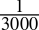. The other scores would change slightly, but this avoids the zeroing-out problem.

# Spam Filter

The canonical machine learning example is building a spam filter. In this section, we will work up a simple spam filter using a Naive Bayesian Classifier and improve it by utilizing a 3-gram tokenization model.

As you have learned before, Naive Bayesian Classifiers can be easily calculated, and operate well under strongly independent conditions. In this example, we will cover the following:

• What the classes look like interacting with each other

• A good data source

• A tokenization model

• An objective to minimize our error

• A way to improve over time

# Setup notes

All of the code we’re using for this example can be found on GitHub.

Ruby is constantly changing, so the README file is the best place to get up to speed on running the examples.

You will have to make sure libxml is installed.

## The Class Diagram

In our example, each email has an object that takes an .eml type text file that then tokenizes it into something the SpamTrainer can utilize for incoming email messages. See Figure 4-2 for the class diagram.

## Data Source

There are numerous sources of data that we can use, but the best is raw email messages marked as either spam or ham. For our purposes, we can use the CSDMC2010 SPAM corpus, which is available on SourceForge.

This data set has 4,327 total messages, of which 2,949 are ham and 1,378 are spam. For our proof of concept, this should work well enough.

## Email Class

The `Email` class has one responsibility, which is to parse an incoming email message according to the RFC for emails. To handle this, we use the mail gem because there’s a lot of nuance in there. In our model, all we’re concerned with is subject and body.

The cases we need to handle are HTML messages, plaintext, and multipart. Everything else we’ll just ignore.

Building this class using test-driven development, let’s go through this step by step.

Starting with the simple plaintext case, we’ll copy one of the example training files from our data set under data/TRAINING/TRAIN_00001.eml to ./test/fixtures/plain.eml. This is a plaintext email and will work for our purposes. Note that the split between a message and header in an email is usually denoted by “\r\n\r\n”. Along with that header information is generally something like “Subject: A Subject goes here.” Using that, we can easily extract our test case, which is:

````require` `'spec_helper'`

`# test/lib/email_spec.rb`

`describe` `Email` `do`
`describe` `'plaintext'` `do`
`let``(``:plain_file``)` `{` `'./test/fixtures/plain.eml'` `}`
`let``(``:plaintext``)` `{` `File``.``read``(``plain_file``)` `}`
`let``(``:plain_email``)` `{` `Email``.``new``(``plain_file``)` `}`

`it` `'parses and stores the plain body'` `do`
`body` `=` `plaintext``.``split``(``"``\n\n``"``)``[``1``.``.``-``1``].``join``(``"``\n\n``"``)`
`plain_email``.``body``.``must_equal` `body`
`end`

`it` `'parses the subject'` `do`
`subject` `=` `plaintext``.``match``(``/^Subject: (.*)\$/``)``[``1``]`
`plain_email``.``subject``.``must_equal` `subject`
`end`
`end`
`end````

Now instead of relying purely on regular expressions, we want to utilize a gem. We’ll use the mail gem, which will handle all of the nitty-gritty details. Making email work for this particular case, we have:

````require` `'forwardable'`

`# lib/email.rb`

`class` `Email`
`extend` `Forwardable`

`def_delegators` `:@mail``,` `:subject`

`def` `initialize``(``filepath``)`
`@filepath` `=` `filepath`
`@mail` `=` `Mail``.``read``(``filepath``)`
`end`

`def` `body`
`@mail``.``body``.``decoded`
`end`
`end````

You’ll notice that we’re using `def_delegators` to delegate `subject` to the `@mail` object. This is just for simplicity’s sake.

Now that we have captured the case of plaintext, we need to solve the case of HTML. For that, we want to capture only the `inner_text`. Knowing that regular expressions are useless for this, we need yet another gem: Nokogiri. Nokogiri will be able to do this for us easily. But first we need a test case, which looks something like this:

````# test/lib/email_spec.rb`

`describe` `Email` `do`
`describe` `'html'` `do`
`let``(``:html_file``)` `{` `'./test/fixtures/html.eml'` `}`
`let``(``:html``)` `{` `File``.``read``(``html_file``)` `}`
`let``(``:html_email``)` `{` `Email``.``new``(``html_file``)` `}`

`it` `"parses and stores the html body's inner_text"` `do`
`body` `=` `html``.``split``(``"``\n\n``"``)``[``1``.``.``-``1``].``join``(``"``\n\n``"``)`
`html_email``.``body``.``must_equal` `Nokogiri``:``:HTML``.``parse``(``body``)``.``inner_text`
`end`

`it` `"stores subject like plaintext does as well"` `do`
`subject` `=` `html``.``match``(``/^Subject: (.*)\$/``)``[``1``]`
`html_email``.``subject``.``must_equal` `subject`
`end`
`end`
`end````

As mentioned, we’re using Nokogiri to calculate the `inner_text`, and we’ll have to use it inside of the `Email` class as well. Now the problem is that we also need to detect the `content_type`. So we’ll add that in:

````# lib/email.rb`

`require` `'forwardable'`

`class` `Email`
`extend` `Forwardable`

`def_delegators` `:@mail``,` `:subject``,` `:content_type`

`def` `initialize``(``filepath``)`
`@filepath` `=` `filepath`
`@mail` `=` `Mail``.``read``(``filepath``)`
`end`

`def` `body`
`single_body``(``@mail``.``body``.``decoded``,` `content_type``)`
`end`

`private`
`def` `single_body``(``body``,` `content_type``)`
`case` `content_type`
`when` `'text/html'`
`Nokogiri``:``:HTML``.``parse``(``body``)``.``inner_text`
`when` `'text/plain'`
`body``.``to_s`
`else`
`''`
`end`
`end`
`end````

At this point, we could add multipart processing as well, but I will leave that as an exercise that you can try out yourself. In the coding repository mentioned earlier in the chapter, you can see the multipart version.

Now we have a working email parser, but we still have to deal with tokenization, or what to extract from the body and subject.

## Tokenization and Context

As Figure 4-3 shows, there are numerous ways to tokenize text, such as by stems, word frequencies, and words. In the case of spam, we are up against a tough problem because things are more contextual. The phrase Buy now sounds spammy, whereas Buy and now do not. Because we are building a Naive Bayesian Classifier, we are assuming that each individual token is contributing to the spamminess of the email.

The goal of the tokenizer we’ll build is to extract words into a stream. Instead of returning an array, we want to yield the token as it happens so that we are keeping a low memory profile. Our tokenizer should also downcase all strings to keep them similar:

````# test/lib/tokenizer_spec.rb`
`require` `'spec_helper'`

`describe` `Tokenizer` `do`
`describe` `'1-gram tokenization'` `do`
`it` `'downcases all words'` `do`
`expectation` `=` `%w[this is all caps]`
`Tokenizer``.``tokenize``(``"THIS IS ALL CAPS"``)` `do` `|``token``|`
`token``.``must_equal` `expectation``.``shift`
`end`
`end`

`it` `'uses the block if given'` `do`
`expectation` `=` `%w[feep foop]`
`Tokenizer``.``tokenize``(``"feep foop"``)` `do` `|``token``|`
`token``.``must_equal` `expectation``.``shift`
`end`
`end`
`end`
`end````

As promised, we do two things in this tokenizer code. First, we lowercase all words. Second, instead of returning an array, we use a block. This is to mitigate memory constraints, as there is no need to build an array and return it. This makes it lazier. To make the subsequent tests work, though, we will have to fill in the skeleton for our tokenizer module like so:

````# lib/tokenizer.rb`

`module` `Tokenizer`
`extend` `self`

`def` `tokenize``(``string``,` `&``block``)`
`current_word` `=` `''`
`return` `unless` `string``.``respond_to?``(``:scan``)`
`string``.``scan``(``/[a-zA-Z0-9_\u0000]+/``)``.``each` `do` `|``token``|`
`yield` `token``.``downcase`
`end`
`end`
`end````

Now that we have a way of parsing and tokenizing emails, we can move on to the Bayesian portion: the SpamTrainer.

## The SpamTrainer

We now need to build the SpamTrainer, which will accomplish three things:

1. Storing training data.

2. Building a Bayesian classifier.

3. Minimizing the false positive rate by testing.

### Storing training data

The first step  we need to tackle is to store training data from a given set of email messages. In a production environment, you would pick something that has persistence. In our case, we will go with storing everything in one big hash.

Remember that most machine learning algorithms have two steps: training and then computation. Our training step will consist of these substeps:

1. Storing a set of all categories

2. Storing unique word counts for each category

3. Storing the totals for each category

So first we need to capture all of the category names; that test would look something like this:

````# test/lib/spam_trainer_spec.rb`

`describe` `SpamTrainer` `do`
`describe` `'initialization'` `do`
`let``(``:hash_test``)` `do`
`{``'spam'` `=>` `'./filepath'``,` `'ham'` `=>` `'./another'``,` `'scram'` `=>` `'./another2'``}`
`end`

`it` `'allows you to pass in multiple categories'` `do`
`st` `=` `SpamTrainer``.``new``(``hash_test``)`
`st``.``categories``.``sort``.``must_equal` `hash_test``.``keys``.``uniq``.``sort`
`end`
`end`
`end````

The solution is in the following code:

````# lib/spam_trainer.rb`

`class` `SpamTrainer`
`def` `initialize``(``training_files``,` `n` `=` `1``)`
`@categories` `=` `Set``.``new`

`training_files``.``each` `do` `|``tf``|`
`@categories` `<<` `tf``.``first`
`end`
`end`
`end````

You’ll notice we’re just using a set to capture this for now, as it’ll hold on to the unique version of what we need. Our next step is to capture the unique tokens for each email. We are using the special category called `_all` to capture the count for everything:

```Subject: One of a kind Money maker! Try it for free!

spam```
````# test/lib/spam_trainer_spec.rb`

`describe` `SpamTrainer` `do`
`let``(``:training``)` `do`
`[[``'spam'``,``'./test/fixtures/plain.eml'``]``,` `[``'ham'``,``'./test/fixtures/small.eml'``]]`
`end`

`let``(``:trainer``)` `{` `SpamTrainer``.``new``(``training``)}`

`it` `'initializes counts all at 0 plus an _all category'` `do`
`st` `=` `SpamTrainer``.``new``(``hash_test``)`
`%w[_all spam ham scram]``.``each` `do` `|``cat``|`
`st``.``total_for``(``cat``)``.``must_equal` `0`
`end`
`end`
`end````

To get this to work, we have introduced a new method called `train!`, which will take the training data, iterate over it, and save it into an internal hash. The following is a solution:

````# lib/spam_trainer.rb`

`class` `SpamTrainer`
`def` `initialize``(``training_files``)`
`setup!``(``training_files``)`
`end`

`def` `setup!``(``training_files``)`
`@categories` `=` `Set``.``new`

`training_files``.``each` `do` `|``tf``|`
`@categories` `<<` `tf``.``first`
`end`

`@totals` `=` `Hash``[``@categories``.``map` `{``|``c``|` `[``c``,` `0``]``}``]`
`@totals``.``default` `=` `0`
`@totals``[``'_all'``]` `=` `0`

`@training` `=` `Hash``[``@categories``.``map` `{``|``c``|` `[``c``,` `Hash``.``new``(``0``)``]``}``]`
`end`

`def` `total_for``(``category``)`
`@totals``.``fetch``(``category``)`
`end`

`def` `train!`
`@to_train``.``each` `do` `|``category``,` `file``|`
`write``(``category``,` `file``)`
`end`
`@to_train` `=` `[]`
`end`

`def` `write``(``category``,` `file``)`
`email` `=` `Email``.``new``(``file``)`

`logger``.``debug``(``"``#{``category``}`` ``#{``file``}``"``)`

`@categories` `<<` `category`
`@training``[``category``]` `||=` `Hash``.``new``(``0``)`

`Tokenizer``.``unique_tokenizer``(``email``.``blob``)` `do` `|``token``|`
`@training``[``category``][``token``]` `+=` `1`
`@totals``[``'_all'``]` `+=` `1`
`@totals``[``category``]` `+=` `1`
`end`
`end`
`end````

Now we have taken care of the training aspect of our program but really have no clue how well it performs. And it doesn’t classify anything. For that, we still need to build our classifier.

### Building the Bayesian classifier

To refresh your memory, Bayes’ theorem is:Score(Spam, W1, W2, …, Wn) = P(Spam)P(W1 | Spam)P(W2 | Spam) … P(Wn | Spam)

which is then divided by some normalizing constant, Z.

Our goal now is to build the methods `score`, `normalized_score`, and `classify`. The `score` method will just be the raw score from the preceding calculation, while `normalized_score` will fit the range from 0 to 1 (we get this by dividing by the total sum, Z).

The `score` method’s test  is as follows:

````# test/lib/spam_trainer_spec.rb`

`describe` `SpamTrainer` `do`
`describe` `'scoring and classification'` `do`
`let` `(``:training``)` `do`
`[`
`[``'spam'``,``'./test/fixtures/plain.eml'``]``,`
`[``'ham'``,``'./test/fixtures/plain.eml'``]``,`
`[``'scram'``,``'./test/fixtures/plain.eml'``]`
`]`
`end`

`let``(``:trainer``)` `do`
`SpamTrainer``.``new``(``training``)`
`end`

`let``(``:email``)` `{` `Email``.``new``(``'./test/fixtures/plain.eml'``)` `}`

`it` `'calculates the probability to be 1/n'` `do`
`scores` `=` `trainer``.``score``(``email``)``.``values`

`assert_in_delta` `scores``.``first``,` `scores``.``last`

`scores``.``each_slice``(``2``)` `do` `|``slice``|`
`assert_in_delta` `slice``.``first``,` `slice``.``last`
`end`
`end`
`end`
`end````

Because the training data is uniform across the categories, there is no reason for the score to differ across them. To make this work in our `SpamTrainer` object, we will have to fill in the pieces like so:

````# lib/spam_trainer.rb`

`class` `SpamTrainer`
`#def initialize`
`#def total_for`
`#def train!`
`#def write`

`def` `score``(``email``)`
`train!`

`unless` `email``.``respond_to?``(``:blob``)`
`raise` `'Must implement #blob on given object'`
`end`

`cat_totals` `=` `totals`

`aggregates` `=` `Hash``[``categories``.``map` `do` `|``cat``|`
`[`
`cat``,`
`Rational``(``cat_totals``.``fetch``(``cat``)``.``to_i``,` `cat_totals``.``fetch``(``"_all"``)``.``to_i``)`
`]`
`end``]`

`Tokenizer``.``unique_tokenizer``(``email``.``blob``)` `do` `|``token``|`
`categories``.``each` `do` `|``cat``|`
`r` `=` `Rational``(``get``(``cat``,` `token``)` `+` `1``,` `cat_totals``.``fetch``(``cat``)``.``to_i` `+` `1``)`
`aggregates``[``cat``]` `*=` `r`
`end`
`end`

`aggregates`
`end`
`end````

This test does the following:

• Trains the model if it’s not already trained (the `train!` method handles this).

• For each token of the blob of an email we iterate through all categories and calculate the probability of that token being within that category. This calculates the Naive Bayesian score of each without dividing by Z.

Now that we have `score` figured out, we need to build a `normalized_score`  that adds up to 1. Testing for this, we have:

````# test/lib/spam_trainer_spec.rb`

`describe` `SpamTrainer` `do`
`it` `'calculates the probability to be exactly the same and add up to 1'` `do`
`trainer``.``normalized_score``(``email``)``.``values``.``inject``(``&``:+``)``.``must_equal` `1`
`trainer``.``normalized_score``(``email``)``.``values``.``first``.``must_equal` `Rational``(``1``,``3``)`
`end`
`end````

and subsequently on the `SpamTrainer` class we have:

````# lib/spam_trainer.rb`

`class` `SpamTrainer`
`#def initialize`
`#def total_for`
`#def train!`
`#def write`
`#def score`

`def` `normalized_score``(``email``)`
`score` `=` `score``(``email``)`
`sum` `=` `score``.``values``.``inject``(``&``:+``)`

`Hash``[``score``.``map` `do` `|``cat``,` `aggregate``|`
`[``cat``,` `(``aggregate` `/` `sum``)``.``to_f``]`
`end``]`
`end`
`end````

### Calculating a classification

Because we now have a score, we need to calculate a classification for the end user to use. This classification should take the form of an object that returns `guess` and `score`. There is an issue of tie breaking here.

Let’s say, for instance, we have a model that has turkey and tofu. What happens when the scores come back evenly split? Probably the best course of action is to go with which is more popular, whether it be turkey or tofu. What about the case where the probability is the same? In that case, we can just go with alphabetical order.

When testing for this, we need to introduce a preference order—that is the occurrence of each category. A test for this would be:

````# test/lib/spam_trainer_spec.rb`

`describe` `SpamTrainer` `do`
`describe` `'scoring and classification'` `do`
`it` `'sets the preference based on how many times a category shows up'` `do`
`expected` `=` `trainer``.``categories``.``sort_by` `{``|``cat``|` `trainer``.``total_for``(``cat``)` `}`

`trainer``.``preference``.``must_equal` `expected`
`end`
`end`
`end````

Getting this to work is trivial and would look like this:

````# lib/spam_trainer.rb`

`class` `SpamTrainer`
`#def initialize`
`#def total_for`
`#def train!`
`#def write`
`#def score`
`#def normalized_score`

`def` `preference`
`categories``.``sort_by` `{``|``cat``|` `total_for``(``cat``)` `}`
`end`
`end````

Now that we have preference set up, we can test for our classification being correct. The code to do that is as follows:

````# test/lib/spam_trainer.rb`

`describe` `SpamTrainer` `do`
`describe` `'scoring and classification'` `do`
`it` `'gives preference to whatever has the most in it'` `do`
`score` `=` `trainer``.``score``(``email``)`
`preference` `=` `trainer``.``preference``.``last`
`preference_score` `=` `score``.``fetch``(``preference``)`

`expected` `=` `SpamTrainer``:``:Classification``.``new``(``preference``,` `preference_score``)`

`trainer``.``classify``(``email``)``.``must_equal` `expected`
`end`
`end`
`end````

Getting this to work in code again is simple:

````# lib/spam_trainer.rb`

`class` `SpamTrainer`
`Classification` `=` `Struct``.``new``(``:guess``,` `:score``)`
`#def initialize`
`#def total_for`
`#def train!`
`#def write`
`#def score`
`#def preference`
`#def normalized_score`

`def` `classify``(``email``)`
`score` `=` `score``(``email``)`
`max_score` `=` `0``.``0`
`max_key` `=` `preference``.``last`
`score``.``each` `do` `|``k``,``v``|`
`if` `v` `>` `max_score`
`max_key` `=` `k`
`max_score` `=` `v`
`elsif` `v` `==` `max_score` `&&` `preference``.``index``(``k``)` `>` `preference``.``index``(``max_key``)`
`max_key` `=` `k`
`max_score` `=` `v`
`else`
`# Do nothing`
`end`
`end`
`throw` `'error'` `if` `max_key``.``nil?`
`Classification``.``new``(``max_key``,` `max_score``)`
`end`
`end````

## Error Minimization Through Cross-Validation

At this point, we need to measure how well our model works. To do so, we need to take the data that we downloaded earlier and do a cross-validation test on it. From there, we need to measure only false positives, and then based on that determine whether we need to fine-tune our model more.

### Minimizing false positives

Up until this point, our goal with making models has been to minimize error. This error could be easily denoted as the count of misclassifications divided by the total classifications. In most cases, this is exactly what we want, but in a spam filter this isn’t what we’re optimizing for. Instead, we want to minimize false positives. False positives, also known as Type I errors, are when the model incorrectly predicts a positive when it should have been negative.

In our case, if our model predicts spam when in fact the email isn’t, then the user will lose her emails. We want our spam filter to have as few false positives as possible. On the other hand, if our model incorrectly predicts something as ham when it isn’t, we don’t care as much.

Instead of minimizing the total misclassifications divided by total classifications, we want to minimize spam misclassifications divided by total classifications. We will also measure false negatives, but they are less important because we are trying to reduce spam that enters someone’s mailbox, not eliminate it.

To accomplish this, we first need to take some information from our data set, which we’ll cover next.

### Building the two folds

Inside the spam email training data is a file called keyfile.label. It contains information about whether the file is spam or ham. Inside our cross-validation test, we can easily parse the file using the following code:

````# test/cross_validation_spec.rb`

`describe` `'Cross Validation'` `do`
`def` `self``.``parse_emails``(``keyfile``)`
`emails` `=` `[]`
`File``.``open``(``keyfile``,` `'rb'``)``.``each_line` `do` `|``line``|`
`label``,` `file` `=` `line``.``split``(``/\s+/``)`
`emails` `<``​``<` `Email``.``new``(``filepath``,` `label``)`
`end`
`emails`
`end`

`def` `self``.``label_to_training_data``(``fold_file``)`
`training_data` `=` `[]`
`st` `=` `SpamTrainer``.``new``(``[]``)`

`File``.``open``(``fold_file``,` `'rb'``)``.``each_line` `do` `|``line``|`
`label``,` `file` `=` `line``.``split``(``/\s+/``)`
`st``.``write``(``label``,` `file``)`
`end`

`st`
`end`

`def` `self``.``validate``(``trainer``,` `set_of_emails``)`
`correct` `=` `0`
`false_positives` `=` `0``.``0`
`false_negatives` `=` `0``.``0`
`confidence` `=` `0``.``0`

`set_of_emails``.``each` `do` `|``email``|`
`classification` `=` `trainer``.``classify``(``email``)`
`confidence` `+=` `classification``.``score`
`if` `classification``.``guess` `==` `'spam'` `&&` `email``.``category` `==` `'ham'`
`false_positives` `+=` `1`
`elsif` `classification``.``guess` `==` `'ham'` `&&` `email``.``category` `==` `'spam'`
`false_negatives` `+=` `1`
`else`
`correct` `+=` `1`
`end`
`end`

`total` `=` `false_positives` `+` `false_negatives` `+` `correct`

`message` `=` `<``​``<-``EOL`
`False` `Positives``:` `#{false_positives / total}`
`False` `Negatives``:` `#{false_negatives / total}`
`Accuracy``:` `#{(false_positives + false_negatives) / total}`
`EOL`
`message`
`end`
`end````

### Cross-validation and error measuring

From here, we can actually build our cross-validation test, which will read fold1 and fold2 and then cross-validate to determine the actual error rate. The test looks something like this:

````# test/cross_validation_spec.rb`
`describe` `'Cross Validation'` `do`
`describe` `"Fold1 unigram model"` `do`
`let``(``:trainer``)` `{`
`self``.``class``.``label_to_training_data``(``'./test/fixtures/fold1.label'``)`
`}`

`let``(``:emails``)` `{`
`self``.``class``.``parse_emails``(``'./test/fixtures/fold2.label'``)`
`}`

`it` `"validates fold1 against fold2 with a unigram model"` `do`
`skip``(``self``.``class``.``validate``(``trainer``,` `emails``))`
`end`
`end`

`describe` `"Fold2 unigram model"` `do`
`let``(``:trainer``)` `{`
`self``.``class``.``label_to_training_data``(``'./test/fixtures/fold2.label'``)`
`}`

`let``(``:emails``)` `{`
`self``.``class``.``parse_emails``(``'./test/fixtures/fold1.label'``)`
`}`

`it` `"validates fold2 against fold1 with a unigram model"` `do`
`skip``(``self``.``class``.``validate``(``trainer``,` `emails``))`
`end`
`end`
`end````

When we run the command `ruby test/cross_validation_spec.rb`, we get the following results:

```WARNING: Could not parse (and so ignoring) 'From spamassassin-devel-admin@lists.sourceforge.net Fri Oct 4 11:07:38 2002'
Parsing emails for ./test/fixtures/fold2.label
WARNING: Could not parse (and so ignoring) 'From quinlan@pathname.com Thu Oct 10 12:29:12 2002'
Done parsing emails for ./test/fixtures/fold2.label
Cross Validation::Fold1 unigram model
validates fold1 against fold2 with a unigram model

False Negative Rate (not so bad): 0.16458622283865001
Error Rate: 0.16828478964401294

WARNING: Could not parse (and so ignoring) 'From quinlan@pathname.com Thu Oct 10 12:29:12 2002'
Parsing emails for ./test/fixtures/fold1.label
WARNING: Could not parse (and so ignoring) 'From spamassassin-devel-admin@lists.sourceforge.net Fri Oct 4 11:07:38 2002'
Done parsing emails for ./test/fixtures/fold1.label
Cross Validation::Fold2 unigram model
validates fold2 against fold1 with a unigram model

False Negative Rate (not so bad): 0.17375231053604437
Error Rate: 0.17929759704251386```

You’ll notice that the false negative rate (classifying an email as ham when it’s actually spam) is much higher than the false positive rate (classifying an email as spam when it’s ham). This is because of Bayes’ theorem! Let’s look at the actual probabilities for ham versus spam in Table 4-3.

Table 4-3. Spam versus ham
Category Email count Word count Probability of email Probability of word

Spam

1,378

231,472

31.8%

36.3%

Ham

2,949

406,984

68.2

63.7%

Total

4,327

638,456

100%

100%

As you can see, ham is more probable, so we will default to that and more often than not we’ll classify something as ham when it might not be. The good thing here, though, is that we have reduced spam by 80% without sacrificing incoming messages.

# Conclusion

In this chapter, we have delved into building and understanding a Naive Bayesian Classifier. As you have learned it, this algorithm is well suited for data that can be asserted to be independent. Being a probablistic model, it works well for classifying data into multiple directions given the underlying score. This supervised learning method is useful for fraud detection, spam filtering, and any other problem that has these types of features.

Get Thoughtful Machine Learning now with the O’Reilly learning platform.

O’Reilly members experience books, live events, courses curated by job role, and more from O’Reilly and nearly 200 top publishers.